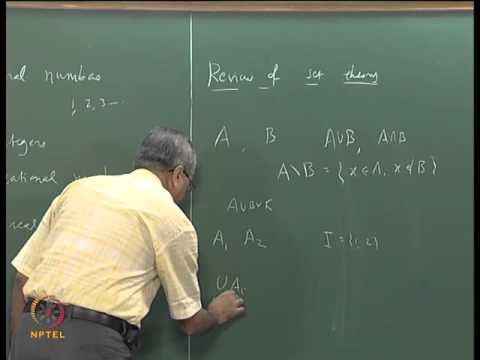NPTEL Courses

# Real Analysis

This course discusses the fundamental concepts in real analysis. Real number system and its order completeness, sequences and series of real numbers. Metric spaces: basic concepts, continuous functions, completeness, contraction mapping theorem, connectedness, intermediate value theorem, compactness, Heine-Borel theorem. Differentiation, Taylor's theorem, Riemann integral, improper integrals, sequences and series of functions, uniform convergence, power series, Weierstrass approximation theorem, equicontinuity, Arzela-Ascoli theorem. (from nptel.ac.in )

Instructor

Mathematics
2016

#### Lecture 01 - Introduction